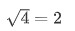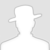FreeBuf.COM网络安全行业门户，每日发布专业的安全资讯、技术剖析。FreeBuf+小程序

Fuzzbook系列（1）：软件的安全性测试2021-01-08 17:53:45

## 简单的测试

```def my_sqrt(x):
"""Computes the square root of x, using the Newton-Raphson method"""
approx = None
guess = x / 2
while approx != guess:
approx = guess
guess = (approx + x / approx) / 2
return approx```

## 了解python

Python通过缩进构造程序，因此函数和`while`主体是通过缩进来定义的；

Python是动态类型的，这意味着变量的类型像`x``approx``guess`在运行时才被确定。

## 运行功能函数

`my_sqrt （4 ）2.0`

`my_sqrt （2 ）1.414213562373095`

## 调试功能函数

```def my_sqrt_with_log(x):
"""Computes the square root of x, using the Newton–Raphson method"""
approx = None
guess = x / 2
while approx != guess:
print("approx =", approx)  # <-- New
approx = guess
guess = (approx + x / approx) / 2
return approx```
`my_sqrt_with_log(9)`
`approx = Noneapprox = 4.5approx = 3.25approx = 3.0096153846153846approx = 3.000015360039322approx = 3.00000000003932143.0`

## 检查功能函数

`my_sqrt （2 ） *  my_sqrt （2 ）`
`1.9999999999999996`

## 自动化测试执行```result = my_sqrt(4)
expected_result = 2.0
if result == expected_result:
print("Test passed")
else:
print("Test failed")```

`Test passed`

`assert my_sqrt(4) == 2`

`EPSILON = 1e-8`
`assert abs(my_sqrt(4) - 2) < EPSILON`

P.S. abs（）求绝对值

`def assertEquals(x, y, epsilon=1e-8):assert abs(x - y) < epsilon`
`assertEquals(my_sqrt(4), 2)assertEquals(my_sqrt(9), 3)assertEquals(my_sqrt(100), 10)`

## 进一步生成测试

`assertEquals(my_sqrt(2) * my_sqrt(2), 2)assertEquals(my_sqrt(3) * my_sqrt(3), 3)assertEquals(my_sqrt(42.11) * my_sqrt(42.11), 42.11)`

`for n in range(1, 1000):assertEquals(my_sqrt(n) * my_sqrt(n), n)`

`my_sqrt()`用100个值测试需要多少时间？让我们来看看。

`import bookutils`
`from Timer import Timer`
```with Timer() as t:
for n in range(1, 10000):
assertEquals(my_sqrt(n) * my_sqrt(n), n)
print(t.elapsed_time())```
`0.022911809000106587`

10,000个值大约需要百分之一秒，因此单次执行`my_sqrt()`需要1/1000000秒或大约1微秒。

`import random`
```with Timer() as t:
for i in range(10000):
x = 1 + random.random() * 1000000
assertEquals(my_sqrt(x) * my_sqrt(x), x)
print(t.elapsed_time())```
`0.02770466199990551`

## 在运行时验证

```def my_sqrt_checked(x):
root = my_sqrt(x)
assertEquals(root * root, x)
return root```

`my_sqrt_checked(2.0)`
`1.414213562373095`

## 系统输入与函数输入

```def sqrt_program(arg):
x = int(arg)
print('The root of', x, 'is', my_sqrt(x))```

`\$ sqrt_program 42`

`sqrt_program("4")`
`The root of 4 is 2.0`

`from ExpectError import ExpectTimeout`
`with ExpectTimeout(1):sqrt_program("-1")`
`Traceback (most recent call last):File "<ipython-input-25-add01711282b>", line 2, in <module>sqrt_program("-1")File "<ipython-input-22-53e8ec8bb3ca>", line 3, in sqrt_programprint('The root of', x, 'is', my_sqrt(x))File "<ipython-input-1-47185ad159a1>", line 7, in my_sqrtguess = (approx + x / approx) / 2File "<ipython-input-1-47185ad159a1>", line 7, in my_sqrtguess = (approx + x / approx) / 2File "ExpectError.ipynb", line 59, in check_timeTimeoutError (expected)`

```def sqrt_program(arg):
x = int(arg)
if x < 0:
print("Illegal Input")
else:
print('The root of', x, 'is', my_sqrt(x))```

`sqrt_program （“ -1” ）`
`Illegal Input`

`from ExpectError import ExpectError`
`with ExpectError():sqrt_program("xyzzy")`
`Traceback (most recent call last):File "<ipython-input-29-8c5aae65a938>", line 2, in <module>sqrt_program("xyzzy")File "<ipython-input-26-ea86281b33cf>", line 2, in sqrt_programx = int(arg)ValueError: invalid literal for int() with base 10: 'xyzzy' (expected)`

```def sqrt_program(arg):
try:
x = float(arg)
except ValueError:
print("Illegal Input")
else:
if x < 0:
print("Illegal Number")
else:
print('The root of', x, 'is', my_sqrt(x))```
`sqrt_program("4")The root of 4.0 is 2.0sqrt_program("-1")Illegal Numbersqrt_program("xyzzy")Illegal Input`

## 测试的极限

`with ExpectError():root = my_sqrt(0)`
`Traceback (most recent call last):File "<ipython-input-34-24ede1f53910>", line 2, in <module>root = my_sqrt(0)File "<ipython-input-1-47185ad159a1>", line 7, in my_sqrtguess = (approx + x / approx) / 2ZeroDivisionError: float division by zero (expected)`

```def my_sqrt_fixed(x):
assert 0 <= x
if x == 0:
return 0
return my_sqrt(x)```

`assert my_sqrt_fixed(0) == 0`

`with ExpectError():root = my_sqrt_fixed(-1)`
`Traceback (most recent call last):File "<ipython-input-37-55b1caf1586a>", line 2, in <module>root = my_sqrt_fixed(-1)File "<ipython-input-35-f3e21e80ddfb>", line 2, in my_sqrt_fixedassert 0 <= xAssertionError (expected)`

## 下一步

# fuzz # 安全测试 # 二进制

+ 收入我的专辑\
• 0 文章数
• 0 评论数
• 0 关注者